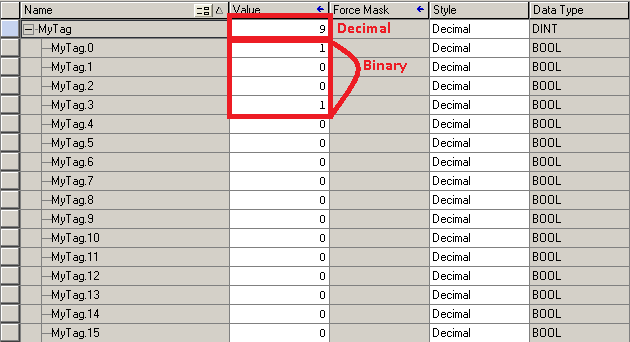# Binary Numbering System 1

## Introduction to the Binary numbering system

Simply put, binary is a base 2 numbering system.    The only numbers available for use to count in binary are 1, and 0.   You can think of this in many ways:  True/False, Yes/No, or High/Low.    Computers work by using the Binary numbering system, because computers only consist of billions of on/off switches.

If we think about our normal every day numbering system, that is called “Decimal”, or Base 10.    The only reason we are used to base 10 is because we have 10 fingers to count on.   When the numbering systems were developed, humans became used to Base 10.  There are 10 “symbols” available for counting (0 through 9).  Remember that leading 0’s do not change the value of a number.  01 is the exact same as 1.    The least significant digit is on the right, because it has less impact on the overall value of the number.   As our numbers get larger, we start adding digits to the right of that number.  We can look at the value of 1000 and the value of 0001.   In the number 1000, the value of 1 has more impact on the value than it does in the number 0001.    As we add numbers to the left, those numbers become more significant.

## Decimal breakdown

Before we understand binary, let’s take a look at this decimal number of 1000.  We need to first change how you think about this number.  Consider the following to understand how numbers are broken down before we try this on other numbering systems.

``` 100 = 1  (Any number to the power of 0 = 1)
101 = 10  (Any number to the power of 1 equals itself)
102 = 100 (10 x 10)
103 = 1000 (10 x 10 x 10)```
```Value:          103 102 101 100
Original number: 1   0   0  0```

Using the Example above, we can see that we have a “1” in the thousands place, so in decimal, we would calculate this as (1 x 1000) + (0 x 100) + (0 x 10) + (0 x 1).   Of course our answer is 1000.

Let’s try this with a different number, such as 2017:

```Value:          103 102 101 100
Original number: 2   0   1  7```

We would calculate this as (2 x 1000) + (0 x 100) + (1 x 10) + (7 x 1)…   The answer is 2017.

## Binary Breakdown

Now that you understand how this works in Decimal, let’s convert a binary number.   Binary is a base 2 numbering system.

``` 20 = 1  (Any number to the power of 0 = 1)
21 = 10  (Any number to the power of 1 equals itself)
22 = 100 (2 x 2)
23 = 8(2 x 2 x 2)```
```Value:           23  2 2 21  20
Original number: 1   0   0  0```

Here we have (1 x 8) + (0 x 4) + (0 x 2) + (0 x 1)  This number equals 8.  Let’s try another one.

```Value:           23 2 2 21 20
Original number: 1  0  0  1```

In this instance, we have (1 x 8) + (0 x 4) + (0 x 2) + (0 x 1) = 9

Let’s look at this on a ControlLogix tag:Notice that “MyTag” is a Double Integer (DINT).   A DINT consists of 32 bits (showing 16 here).   We can look at this number as an Integer, or we can expand the tag to break it down into Binary.   We see the value of 1001 in binary is the value of “9” in decimal.  When we look at a tag, we need to ask ourselves what we want to look at.   If we want to look at it as a number (such as a temperature), then we look at the number at the DINT level.   If the tag is used for individual discrete (on/off) devices, then we can expand the tag to see which individual bits are turned on.